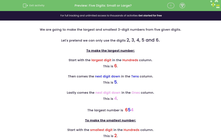# Make the smallest and largest number

In this worksheet, students will be given five digits from which they must identify the smallest and largest three-digit numbers.Key stage:  KS 2

Curriculum topic:   Number: Number and Place Value

Curriculum subtopic:   Order/Compare Numbers to 1000

Difficulty level:#### Worksheet Overview

In this activity, we are going to make the largest and smallest 3-digit numbers from five given digits.

Let's pretend we can only use the digits 2, 3, 4, 5 and 6.

To make the largest number:

This is 6.

Then comes the next largest digit down in the tens column.

This is 5.

Lastly, comes the next largest digit in the ones column.

This is 4.

The largest number is  654

Did you notice that we didn't need to use the two smallest numbers, 2 and 3, to make this number?

To make the smallest number:

This is 2.

Then comes the next largest digit in the tens column.

This is 3.

Lastly, comes the next largest digit  in the ones column.

This is 4.

The smallest number is  234.

Did you notice that we didn't need to use the two largest numbers, 5 and 6 this time, to make this number?

Let's try an example question together using the same method.

Example

Using any 3 of the digits from:

68671

make the smallest and largest 3-digit numbers that you can make.

First, we should put the digits in order:

1, 6, 6, 7, 8

To find the smallest 3-digit number we do the following:

1 is the smallest digit.

6 is the next largest digit.

6 is the next largest digit, even though it is the same as the last one.

So, the smallest number will be 166.

Now to find the largest possible 3-digit number:

8 is the largest digit.

7 is the next digit down.

6 is the next digit down.

So, the largest number will be 876.

Now that you've seen a good method, it's time to try some questions on your own.